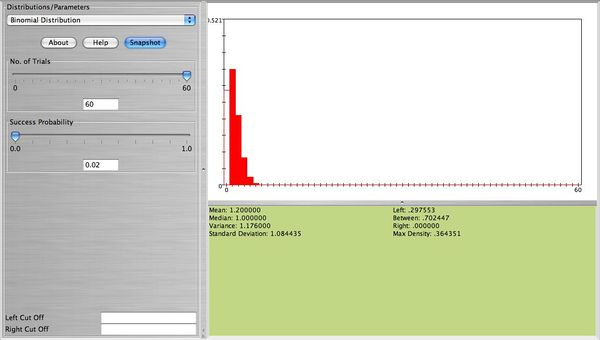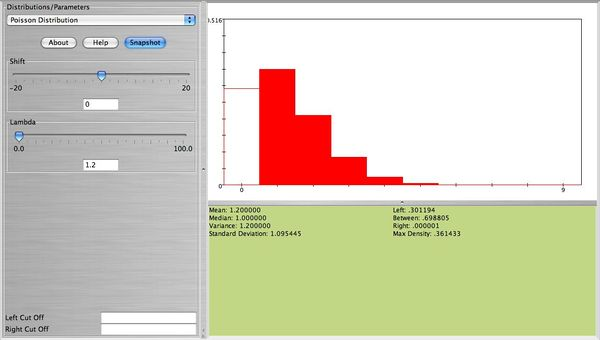# AP Statistics Curriculum 2007 Limits Poisson2Bin

## General Advance-Placement (AP) Statistics Curriculum - Poisson as Approximation to Binomial Distribution

### Poisson as Approximation to Binomial Distribution

$np < 10$ $n \geq 20$ and $$p \leq 0.05$$.

### Examples

The Binomial distribution can be approximated well by Poisson when $$n$$ is large and $$p$$ is small with $$np < 10$$, as stated above. This is true because $$\lim_{n \rightarrow \infty} {n \choose x} p^x(1-p)^{n-x}=\frac{\lambda^x e^{-\lambda}}{x!}$$, where $$\lambda=np$$. Here is an example. Suppose $$2\%$$ of a certain population have Type AB blood. Suppose 60 people from this population are randomly selected. The number of people $$X$$ among the 60 that have Type AB blood follows the Binomial distribution with $$n=60, p=0.02$$. The figure below represents the distribution of $$X$$. This figure also shows $$P(X=0)$$.• Note: This distribution can be approximated well with Poisson with $$\lambda=np=60(0.02)=1.2$$. The figure below is approximately the same as the figure above (the width of the bars is not important here. The height of each bar represents the probability for each value of $$X$$ which is about the same for both distributions).